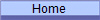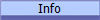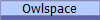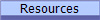﻿ Comp310 Lec19

# Lec19:  Java GenericsPlease also refer to the supplemental PowerPoint slides for today's material, written by ex-Rice graduate student Anupam Chanda for Comp212:

## The Continued Push for Abstraction

So far, we've used abstract classes and polymorphism extensively to model a high level of abstraction in our software systems.  But is there more that can be done?

For instance, we often use the class Object to represent "any class".    But we can run into problems with type safety in doing this.  Consider the following example, where we are trying to model a "box" that hold objects:

```public class OldBox {
Object data;

public OldBox(Object data) {
this.data = data;
}

public Object getData() {
return data;
}
}
```

Using an instance of this class requires that we use a cast:

```OldBox intBox = new OldBox(42);
int x = (Integer) intBox.getData();```

Since a Box holds an Object , we can do the same thing for a box with a String datum:

```OldBox strBox = new OldBox("Howdy");
String s = (String) strBox.getData();```

But the compiler has no idea exactly what type of object is being held in the box at any given moment, so the following line of  code compiles just fine:

```int y = (Integer) strBox.getData();
intBox = strBox;```

Shortly all your customers are clogging your help lines with "Why am I getting a ClassCastException when I run your code!" (more colorful articulations deleted).

The bottom line here is perhaps we didn't really want to make a box that held any class of data, but rather a box that held a specific class of data where we didn't care what that class was when we defined the behavior of the box.    The difference between those two points of view is subtle but extremely important.   Take the time to understand it.

That is, we really want to define the box with an abstraction of the particular class it is to hold.    Object represents the union of all possible classes, which not what we want here--we want a specific class, abstractly represented.

## Parameterized Classes

Java, starting with version 5.0, has a feature called "parametric polymorphism", or "generics".    On the surface, this looks similar to the templates in C++, but in reality, it is quite different in many aspects.

Consider the following definition of "a box of E":

```public class Box {
E data;

public Box(E data) {
this.data = data;
}

public E getData() {
return data;
}
}
```

The "<E>" after the class name is a parameterization of the class definition using the symbol "E".   That is, E refers to a particular type and that anywhere E appears in the class definition, the particular class type referred to by E should be used.

Notice for instance, that the constructor for Box takes an input of the specific type E, not any type (i.e. subclass of Object).   Likewise, the getData() method returns exactly the type being held, not the superclass Object.

To instantiate a concrete Box<E> with that holds a particular type of data, we simply supply the name of the class we want E to be:

```Box intBox = new Box(42);
int x = intBox.getData() // No cast needed since the exact return type is known!

Box strBox = new Box("Howdy");
String s = strBox.getData() // No cast needed since the exact return type is known!```
The lines of code that used to compile but give us run-time cast errors now won't compile at all:
```String s = (String) intBox.getData();
int y = (Integer) strBox.getData();
intBox = strBox;```

The use of a parameterized class has transformed run-time errors into compile-time errors.   In this particular case, we say that the generics have increased the "type safety" of our system because more erroneous type-related mistakes are caught by the compiler.

Using parameterized classes is particularly useful in defining "container" classes which hold data but don't process that data.  Thus the client wants their abstract behavior to work for holding any specific kind of data that the client desires.  Starting with Java 5.0, all the supplied collections framework classes in Java are defined using generics (see the Java 6 API documentation and the Collections Framework documentation).

Syntax Note:  A class can be parameterized by more than one parameter.   To do so, simply separate the parameters by commas, e.g:

`public class Stuff { ... }`

One can define subclasses of  parameterized classes either by extending a particular type or by extending a parameterized type:

```class IntBox extends
Box { ... }```

or

```class SpecialBox extends
Box { ... }```

It should be noted that the second definition above does mean that SpecialBox<String> is a subclass of Box<String>.  That is,

```Box sb = new
SpecialBox("Yahoo!");```

is a legal assignment.   So, the inheritance relationships for specific parameterized types where the type parameter is explicitly specified, as above, still work as normal.

### Parameterized Classes in Methods

A parameterized class is a type just like any other type (class -- remember that a class definition is really a type definition) in the system.   Thus they can be used in method input types and return types just as any other type, e.g.

```Box aMethod(int i,
Box b) { ... }```

If the class definition is parameterized, that type parameter can be used as a type parameter for any type declaration in that class, e.g.

```public class Box {
E data;

public Box(E data) {
this.data = data;
}

public E getData() {
return data;
}

public void copyFrom(Box b) {
this.data = b.getData();
}
}```

In effect, we've added an infinite number of different types of Boxes to our system by only writing a single class definition!

## Bounded Parameterized Types

Sometimes we don't want to parameterize out class or method with just any old type, but we want to put some restrictions on them.  For instance, suppose we want a box that holds particular kinds of numbers, called MathBox.   We can't use a regular Box<E> because E could be anything.    What we want to say is that E must be a subtype of Number.

```public class MathBox extends Box {
public MathBox(E data) {
super(data);
}

public double sqrt() {
return Math.sqrt(getData().doubleValue());
}
}```
The <E extends Number> syntax above means that the type parameter of MathBox must be a type which is a subclass of the Number class.   We say that the type parameter is bounded.   Thus the following declarations are legal:

new MathBox<Integer>(5)

new MathBox<Double>(32.1)

But the following is illegal because String is not a subclass of Number.

new MathBox<String>("No good!")

Note that inside of a parameterized class, the type parameter serves as a valid type, so it can be used in a bounded type parameter situation just as any other type can, e.g.

```public class OuterClass{
private class InnerClass{
...
}

...
}```

Syntax note:  The <A extends B> syntax applies even if B is an interface because 1) it doesn't matter operationally if B is a class or an interface and 2) if B is a type parameter, the compiler doesn't know a priori whether it is a class or an interface.

Since Java allows multiple inheritance in the form of implementing multiple interfaces, it is possible that multiple bounds are necessary to specify a type parameter.    To express this situation, one uses the following syntax:

<T extends A & B & C & ...>

For instance:

```interface A {
...
}

interface B {
...
}

class MultiBounds {
...
}```

## Parameterized Methods

Parameterizing just the class is not always enough to insure the type safety of a method.   Sometimes an additional parameterization of the method itself is required.  For instance, suppose you  want the return type of a method to match the input parameter type, neither of which is related to the class's type parameter:

```public  T aMethod(T x) {
...code possibly involving T...
}```

The <T> at the front of the method signature is a type parameter just for that one method.  The above method signature says that whatever type aMethod() is called with as an input parameter, that is the type of aMethod()'s return value.    Notice that it doesn't make sense to use a type parameter in a method definition unless that type is referenced more than once in the method.

For example, we could add the following parameterized method to Box<E> to see if another box holds superclasses what this Box holds (E):

```  public  boolean holdsSubClassOf(Box b) {
T d_inp = b.getData();
E d_this = getData();
return d_inp.getClass().isAssignableFrom(d_this.getClass());
}
```

## Upper Bounded Wildcards in Parameterized Types

We've definitely made progress here, but we start to run into some new issues when we start to do some things that seem "normal".   For instance, one would think the following would be reasonable:

Box<Number> numBox = new Box<Integer>(31);

But the compiler comes back with an "Incompatible Type" error message because Box<Number> and Box<Integer> are not fundamentally related to each other.

This is not an unreasonable situation however.   We might have code that processes Box<Number> objects, which means that by regular "run-time" or "ad-hoc" polymorphism (the usual polymorphism we've been using all along), the data held in a Box<Integer> object should work just fine in our system.

The problem is that Box<Number> numBox defines an invariant type relationship between numBox and any object to which it refers, where the referenced object's type parameter must match  numBox's type parameter exactly.

What we want is a covariant type relationship where the referenced object's type parameter is a subclass of numBox's type parameter.   This is the relationship in our failed attempt above.

To be more specific, the type of numBox we desire is "a Box of any type which extends Number".    The Java syntax to express this is

Box<? extends Number> numBox = new Box<Integer>(31);

For example, we could now rewrite our copyFrom() method above so that it would accept a Box of any type that is covariant to Box<E> since such a box would contain data that was a subclass of E and thus be able to be stored in a Box<E> object.

```public void copyFrom(Box<? extends E> b) {
this.data = b.getData(); // b.getData() is a subclass of this.data
}```

This enhancement greatly increases the utility of the copyFrom() method because it is now not limited to only inputs of type Box<E> but will now work with any compatible Box object.

The type parameterization <? extends E> is called an "upper bounded wildcard" because it defines a type that could be any type so long as it is bounded by the superclass E.

Question:   Why don't we have to worry about this if we were to write a setter method for Box<E>?   That is, why is it sufficient to write the following?

```public void setData(E data) {
this.data = data;
}```

## Lower Bounded Wildcards in Parameterized Types

Sometime however, we have the opposite problem from above.   Let's look at an example:  Suppose we wish to write a method similar to our copyFrom() method above called copyTo()such that it copies the data the opposite direction, i.e. from the host object to the given object:

```public void copyTo(Box<E> b) {
b.data = this.data();
}```

The above code works fine so long as you are copying from one Box to another of exactly the same type (invariant type relationship), e.g. both are Box<Integer> or both are Box<String>. But operationally, b could be a Box of any type that is a superclass of E and the copying would be type safe.  This is a contravariant type relationship between Box<E> amd the input parameter to the copyTo() method where the type parameter of the object referenced by the variable b  is a superclass of E.

To express this, we write

```public void copyTo(Box<? super E> b) {
b.data = this.data(); // b.data is a superclass of this.data
}```

The type parameterization <? super E> is called a "lower bounded wildcard" because it defines a type that could be any type so long as it is bounded by the subclass E.

Once again, we have greatly increased the utility of the copyTo() method because it is now not limited to only inputs of type Box<E> but will now work with any compatible Box object.

Upper and lower bounded wildcards will prove indispensable when implementing the visitor pattern on generic (parameterized) classes.

## Unbounded Wildcards in Parameterized Types

The ? type parameter alone represents what is called a "bivariant type relationship" where the referenced object's type parameter could be either a subclass or a superclass of the variable's type parameter.    In other words, any type parameter will work.   The ? type parameter is called an "unbounded wildcard" here.

Thus, the following statements are all  legal:

Box<?>  b1 = new Box<Integer>;

Box<?>  b2 = new Box<String>;

b1 = b2;

Question:   What is the difference between Box<?> and Box<Object>?

Unbounded wildcards are useful when writing code that is completely independent of the parameterized type.

### Wildcard Capture

Notice that the compiler can figure out exactly what type b1 is above because the right hand side of the equals sign unequivocally defines the type parameter.   This "capturing" of the type information by the wildcard means that

1. The type on the left hand side doesn't need to be specified.
2. The compiler can do additional type checks because it knows the type of b1 .

Wildcard capture enables robust, type safe code to be written with a minimum of concrete specifications.

A Zip file with the code from today's class can be found here: generics.zip